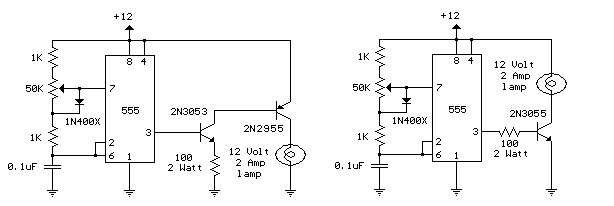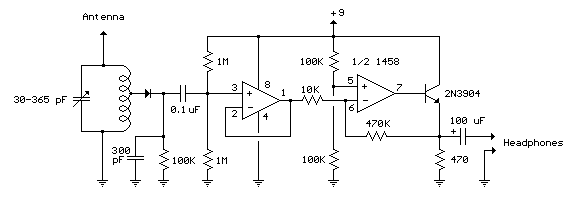### Parallel Port Relay Interface

Here is an example of controlling a relay from the PC's parallel printer port (LPT1 or LPT2). The 7.5K resistor is connected to one of the data output lines (D0-D7) and a common connection is made from the negative side of the power supply to one of the port ground pins (18-25). Data is inverted by the 3904 transistor so that writing a "0" to the base address will energize any relays connected to D0-D7 and a "255" will reset them. You may need to try all three base addresses to determine the correct address for the port you are using but LPT1 is usually at Hex 0378. The QBasic "OUT" command can be used to send data to the port. OUT, &H0378,0 sets D0-D7 low and OUT, &H378,255 sets D0-D7 high.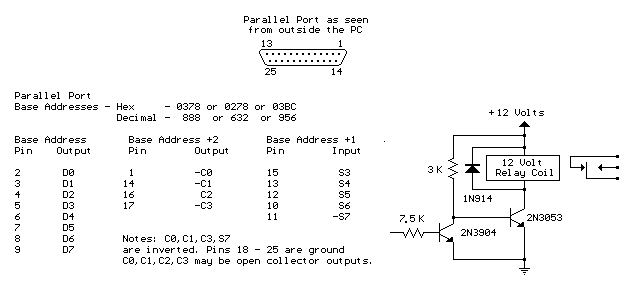### Reading Data From The Parallel Port

The diagram below shows 5 switches connected to the 5 input lines of the parallel port. An external 5 volt power supply is used to provide high logic levels to the input pins when the switches are open. Three 1.5 volt batteries in series can be used to obtain 4.5 volts which is close enough. The 330 ohm resistors in series with the port connections provide some protection in case a connection is made to the wrong pin. If you are sure of the connections, the 330 ohm resistors can be left out and the switches connected directly to the input pins. The negative side of the power supply should be connected to the ground point, or any pin from 18 to 25.

The following short QBasic program can be used to read the state of the switches. QBASIC.EXE can be found in the "OLDMSDOS" directory of the Windows 95/98 CD Rom. Note that there are three possible printer port address that correspond to LPT1, LPT2 and LPT3 and LPT1 is usually the one to use which is at address decimal 889. The program waits for the user to press the enter key before reading the state of the 5 input lines. The state of the 5 lines is received as a single 8 bit number between 0-255 which is stored as the value of (V). Each switch input represents a decimal value of 8,16,32,64 and 128 which correspond to pins 15,13,12,10 and 11. The last 3 bits (1,2 and 4) are not used and should return a high level, so the value received with all switches open should be 1+2+4+8+16+32+64=127. If a switch is closed and the input is at ground, the value will be 0 except for pin 11 which is inverted and yields a value of 128 and 0 when high, so the value received when all switches are closed should be 1+2+4+128=135.
-----------------------------------------------------------------------

CLS
DEFINT A-Z
PRINT "Press the enter key to read printer port pins (15,13,12,10,11)"
PRINT "A (0) reading indicates the pin is at ground level, (1) indicates"
PRINT "the pin is at a high level or unterminated."
INPUT A\$
PRINT V
P11 = 1
IF V > 127 THEN P11 = 0: V = V - 128
IF V > 63 THEN P10 = 1: V = V - 64
IF V > 31 THEN P12 = 1: V = V - 32
IF V > 15 THEN P13 = 1: V = V - 16
IF V > 7 THEN P15 = 1
PRINT
PRINT "Pin 15 ="; P15
PRINT "Pin 13 ="; P13
PRINT "Pin 12 ="; P12
PRINT "Pin 10 ="; P10
PRINT "Pin 11 ="; P11
END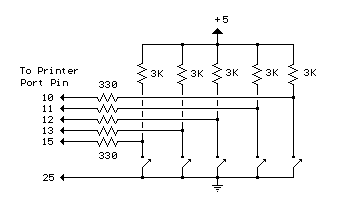### Micro Power AM Broadcast Transmitter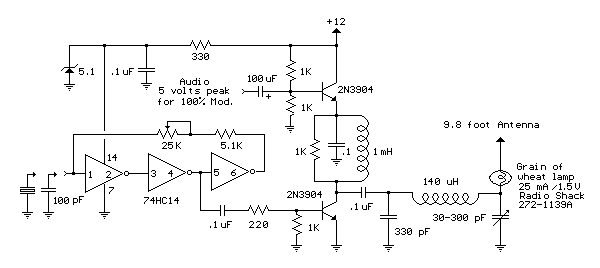### FM Beacon Broadcast Transmitter (88-108 MHz)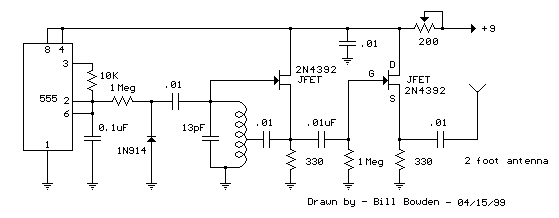### 12 Volt Lamp Dimmer

Here is a 12 volt / 2 amp lamp dimmer that can be used to dim a standard 25 watt automobile brake or backup bulb by controlling the duty cycle of a astable 555 timer oscillator. When the wiper of the potentiometer is at the uppermost position, the capacitor will charge quickly through both 1K resistors and the diode, producing a short positive interval and long negative interval which dims the lamp to near darkness. When the potentiometer wiper is at the lowermost position, the capacitor will charge through both 1K resistors and the 50K potentiometer and discharge through the lower 1K resistor, producing a long positive interval and short negative interval which brightens the lamp to near full intensity. The duty cycle of the 200 Hz square wave can be varied from approximately 5% to 95%. The two circuits below illustrate connecting the lamp to either the positive or negative side of the supply.